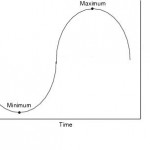Oct
20

## Find minimum and maximum using AWKLet’s assume you want to find the minimum and/or maximum of a column of numbers in a given file using AWK.

Assume we have an input file foo with f.ex. line number in first column and in the second (\$2) and third column (\$3 in awk) we have the values of interest.

File: foo

1 2 40
2 3 23
3 15 -12
4 8 -1
5 11 0

Use the following awk commando find minimum and maximum of the columns of interest:

awk ‘NR == 1 {max=\$2 ; min=\$2} \$2 >= max {max = \$2} \$2 <= min {min = \$2} END { print "Min: "min,"Max: "max }' foo

or

awk ‘BEGIN{ max = -999999999 ; min = 9999999999 } \$2 > max {max = \$2} \$2 < min {min = \$2} END{ print "Min: ",min, "Max: ",max }' foo

The result will be for column 2:

Min: 2 Max: 15

and for column 3:

Min: -12 Max: 40

#### Standard deviation

Here you may see how to calculate the standard deviation using AWK.

#### Average or arithmetic mean

Here you may see how to calculate the average or arithmetic mean using AWK.

#### 1 comment

1.##### hyewon.lee says:

hi
i found ur homepage accidently 😉

but ur a lot of information is very useful to me !!

thanks touch me using “awk” and other ~

anyway, what is ur major ??

i’m major seismology in KOREA
here is my homepage join me ~

have a nice day!!